# To Latch or not to Latch?

Yesterday I explained to a colleague the effect of the Inport block option Latch input for feedback signals of function-call subsystem outputs . I thought it would be interesting to share here.

The Problem

In the following model, inside the calib function-call subsystem, the Count signal is connected to a Unit Delay block.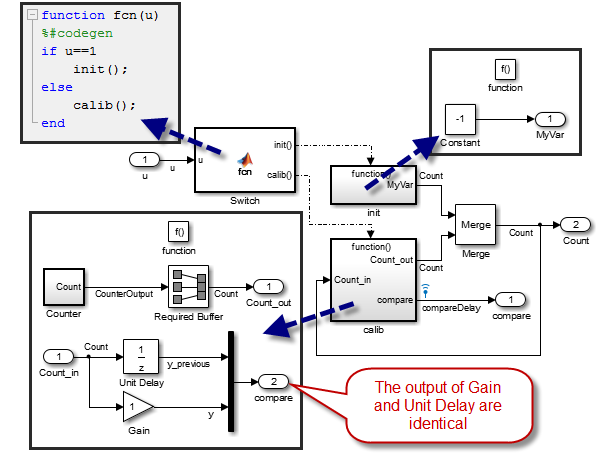When logging data, we can see that the input and output of the Unit Delay are identical, as if the signal was not delayed!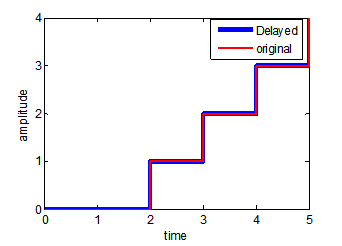Even though this might seem strange, this behavior is expected. Let's see why.

The simulation loop

To understand what is happening, you first need to be familiar with the order into which the block methods are executed within the simulation loop. To really understand the simulation loop I recommend the following documentation pages:

In our case, we have a model that is fixed-step and discrete, so the simulation loop is very simple and looks like this: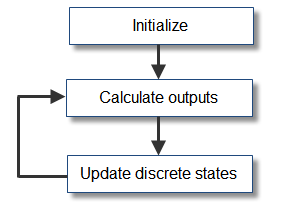The important thing to understand is that Simulink executes the Outputs method of all blocks, and then the Update method of all blocks.

The execution order

The second thing we need to know is the order in which blocks are executed. For that, we display the block sorted order.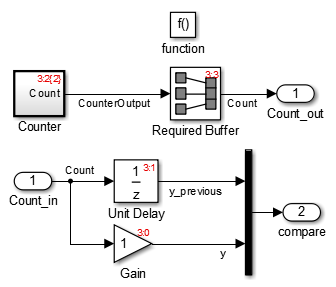When you display the sorted order, you can see red numbers displayed on the block. The first number is the number of the system, so the function-call subsystem in this model is system number 3. The second number is the order of the block in this system. In this case, the Gain is the first (3:0), Unit Delay the second (3:1), Counter the third (3:2), etc.

The effect of the latch

To see the effect of latching, I generated code for this model and added comments to highlight the fact that the feedback loop causes the input signal to change immediately as the output is written: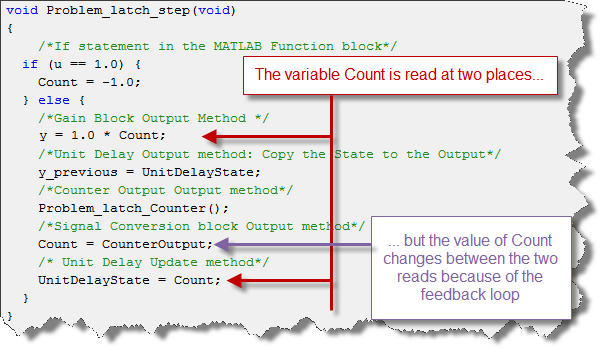Such feedback loops break the assumptions on which the Output-Update logic is built... and can make results difficult to interpret. This is why the Latch is available.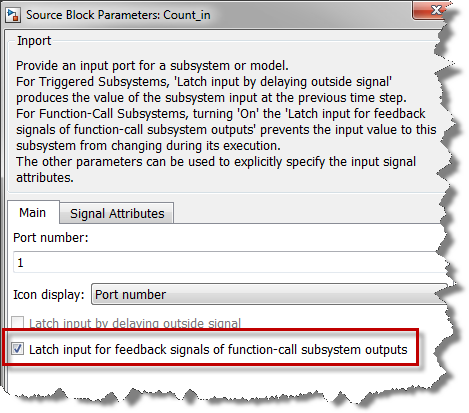With the latch, a copy of the input signal is made at the beginning of the step and this copy is used for the computations: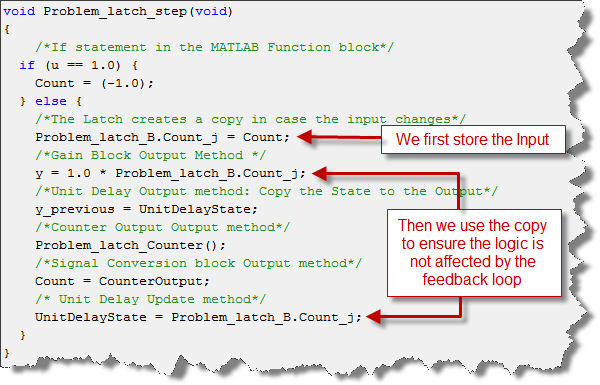Conclusion

In conclusion, we can say that introducing the latch is safer, but costs an additional copy. If you know that your function-call subsystem might be involved in a direct feedback loop, use Inport Latching to avoid surprises.

Now it's you turn

Are you latching the inputs of your function-call subsystems? Let us know the reason why by leaving a comment here.

|